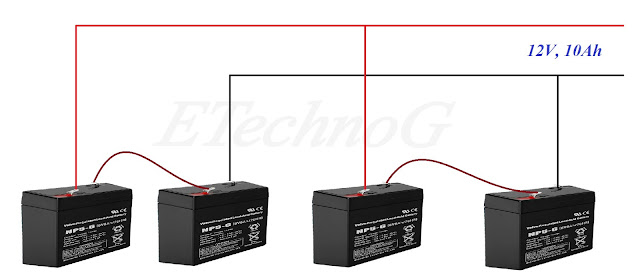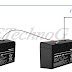## Battery Wiring in Series and Parallel

Before going to make Battery Wiring in Series and Parallel you must know about the rating of a battery.

### Understanding the Rating of Battery:

A battery has the rating 6V, 5Ah. Let's know what is the meaning of 6V, 5Ah.
6V, 5Ah means when you connected a load with the battery if the load draw 5A current then the battery can give power for one hour to the load. If the load draw 2.5A current then the battery can give power for two hours to the load. If the load draw 10A current then the battery can give power for half hours to the load.

### Battery with Series and Parallel Connection :

Now let's design current and voltage capacity. Here we used  6V, 5Ah battery. One thing you must be remembered that when we connect batteries in parallel the effective voltage will be the same as previous but current rating will be increased.
When we connect batteries in series the effective voltage will be increased but the current rating will be the same as previous.

For better understanding see the below examples:

(1) If we want to design 12V, 5Ah rating. So we need two batteries having the rating of  6V, 5Ah. Both batteries are to be connected in series.

(2) If we want to design 6V, 10Ah rating. So we need two batteries having the rating of  6V, 5Ah. Both batteries are to be connected in parallel.

(3) If we want to design 12V, 10Ah rating. So we need four batteries having the rating of  6V, 5Ah. The connection is given below.Batteries in series and parallel connection.

Battery Wiring in Series and Parallel-Full explanation.Reviewed by Author on December 21, 2018 Rating: 5